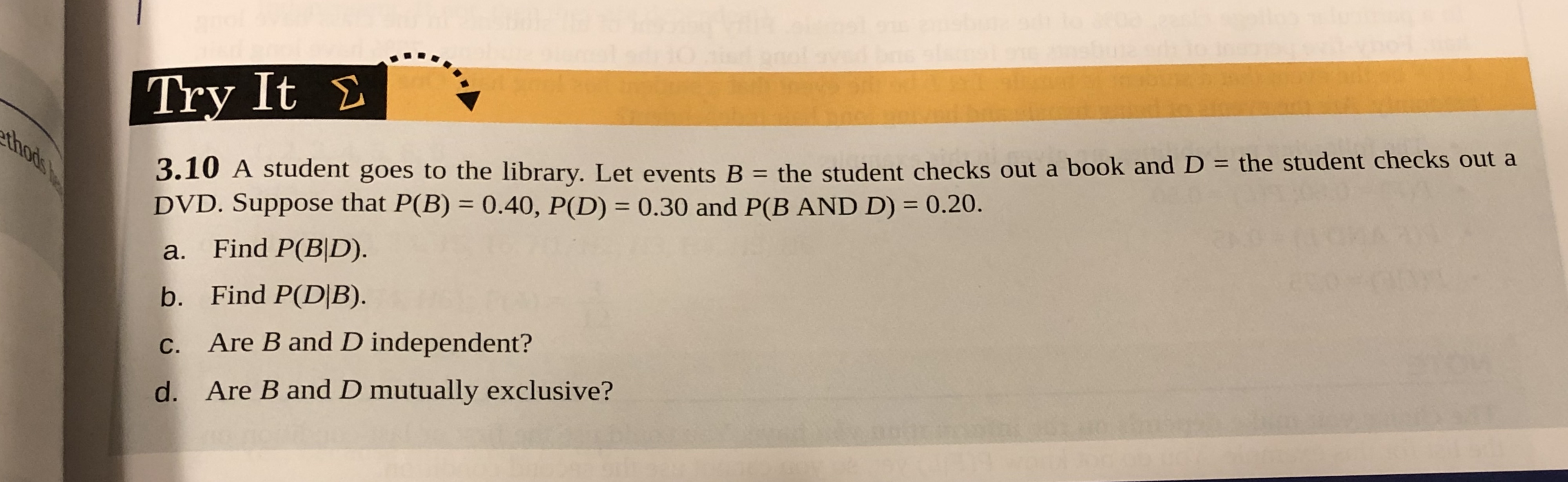# Try It Σ 3.10 A student goes to the library. Let events B - the student checks out a book and D - the student checks out a DVD. Suppose that P(B) 0.40, P(D) 0.30 and P(B AND D)0.20. a. Find P(B|D). b. Find P(DIB) c. Are B and D independent? d. Are B and D mutually exclusive?

Question

A student goes to the library. Let events B= the student checks out a book and D= the student checks out a DVD. Suppose that P(B)= 0.40, P(D)= 0.30 and P(A and B)= 0.20.

A. Find P(B|D).

B. Find P(D|B).

C. Are B and D independent?

D. Are B and D mutually exclusive?help_outlineImage TranscriptioncloseTry It Σ 3.10 A student goes to the library. Let events B - the student checks out a book and D - the student checks out a DVD. Suppose that P(B) 0.40, P(D) 0.30 and P(B AND D)0.20. a. Find P(B|D). b. Find P(DIB) c. Are B and D independent? d. Are B and D mutually exclusive? fullscreen

### Want to see this answer and more?

Experts are waiting 24/7 to provide step-by-step solutions in as fast as 30 minutes!*